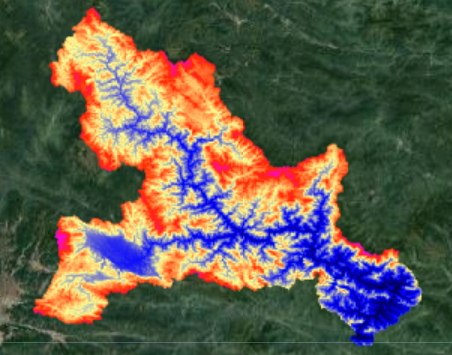# Volume height relation for a reservoir

Calculate the volume height relation for existing or potential reservoirs.

In this exercise we are going to calculate the water level – volume curve of the Ban Ve dam.

Step 1: import the upstream area:

```// get the upstream area of the dam
var BanVEupstream = ee.FeatureCollection('ft:1z6zLTS1z5FlO1jizxY9g7k6-IIAcJvvT-5VxzVeL');
```

The dam is located at the red arrowStep 2: Import the srtm and clip the basin

```// import srtm data
var srtm = ee.Image(&quot;CGIAR/SRTM90_V4&quot;);
// clip ban ve area
var dem = srtm.clip(BanVEupstream);

var viz = {min:0, max:2000, palette:&quot;000000,0000FF,FDFF92,FF2700,FF00E7&quot;};
```Step 3: Add a recent sentinel 2 image for visual interpretation

```// add recent s2 rgb image of reservoir
var img = ee.Image(&quot;COPERNICUS/S2/20160209T034234_20160209T090731_T48QVG&quot;);
```Step 4: Create a list with water levels

```// set list with water levels
var waterlevel = ee.List.sequence(155,220,1);
```

Step 5: Copy paste the function below. This function calculates water depth for different water levels using the digital elevation model.

```// Function to calculate the volume in the reservoir
var calcVolume = function(level,list){

// calculate area inundated
var inundated = srtm.clip(BanVEupstream).lte(ee.Number(level));

// calculate water level in each pixel
var water = inundated.multiply(dem).subtract(ee.Number(level)).multiply(-1)

// calculate area
var area = inundated.multiply(30).multiply(30);

// calculate volume
var volume = area.multiply(water);

// sum the volume
var totalvolume = volume.reduceRegion(ee.Reducer.sum(), BanVEupstream);

// retunr result to list
};
```

Step 6: Apply the function above and put the values in an array

``` // create emtpy list
var first = ee.List([]);

// iterate over water levels
var Volumes = ee.List(waterlevel.iterate(calcVolume, first));

// set x an y values for list
var xValues = ee.Array(Volumes).divide(1000000000);
var yValues = ee.Array(waterlevel);
```

Step 7: Print the data as a chart.

```//  Create the chart
var chart = ui.Chart.array.values(yValues, 0, xValues)
.setOptions({
title: 'Relation Volume Waterlevel',
vAxis: {'title': 'water level (m)'},
hAxis: {'title': 'Volume (billion m3)'},
pointSize: 3,
});

// Print the chart.
print(chart)
```1.Francy Lisboa says:

This is awesome!!!!!!

2.Tanzim Taher says:

I have a query regarding this code:

// calculate area
var area = inundated.multiply(30).multiply(30);

I get confused. If we are using SRTM 90m resolution imagery, then shouldn’t the code be:
// calculate area
var area = inundated.multiply(90).multiply(90);

1.thisearthsite says:

Excellent point. Use this one instead: USGS/SRTMGL1_003

1.tanzimtaher says:

Yep, makes sense!

I tried the SRTM version 3 30 m resolution dataset (“USGS/SRTMGL1_003”). Gives the same results as writing: var area = inundated.multiply(90).multiply(90);

3.tanzimtaher says:

About the calcVolume function, this particular line of code:
// calculate area
var area = inundated.multiply(30).multiply(30);
Since we are using SRTM 90m resolution imagery, shouldn’t the code be:
// calculate area
var area = inundated.multiply(90).multiply(90);

4.amirhossein says: